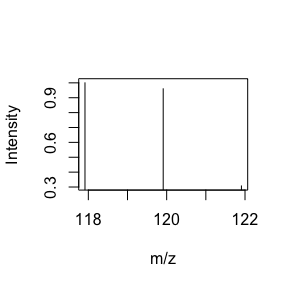# Handling Molecular Formulae

## Introduction

The molecular formula is the simplest way to characterize a molecular compound. It specifies the actual number of atoms of each element contained in the molecule. A molecular formula is represented by the chemical symbol of each constituent element. If a molecule contains more than one atom for a particular element, the quantity is shown as subscript after the chemical symbol. Otherwise, the number of neutrons (atomic mass) that an atom is composed can differ. This different type of atoms are known as isotopes. The number of nuclei is represented as superscripted prefix previous to the chemical element. Generally it is not added when the isotope that characterizes the element is the most occurrence in nature, e.g., $$C_4H_{11}O^2D$$.

## Parsing a Molecule To a Molecular Formula

Front a molecule, defined as conjunct of atoms helding together by chemical bonds, we can simplify it taking only the information about the atoms. The rcdk package provides a parser to translate molecules to molecular formlulas, the get.mol2formula function.

library(rcdk)
## Loading required package: rcdklibs
## Loading required package: rJava
sp <- get.smiles.parser()
molecule <- parse.smiles('N')[]
convert.implicit.to.explicit(molecule)
formula <- get.mol2formula(molecule,charge=0)

Note that the above formula object is a CDKFormula-class. This class contains some attributes that defines a molecular formula. For example, the mass, the charge, the isotopes, the character representation of the molecular formula and the IMolecularFormula jobjRef object.

The molecular mass, charge and string representation for this formula are given by

formula@mass
##  17.02655
formula@charge
##  0
formula@string
##  "H3N"

The isotopes for this formula. It is formed from three attributes. isoto (the symbol expression of the isotope), number (number of atoms for this isotope) and mass (exact mass of this isotope).

formula@isotopes
##      isoto number mass
## [1,] "H"   "3"    "1.007825032"
## [2,] "N"   "1"    "14.003074"

Depending of the circumstances, you may want to change the charge of the molecular formula.

formula <- set.charge.formula(formula, charge=1)

## Initializing a Formula from the Symbol Expression

Other way to create a cdkFormula is from the symbol expression. Thus, setting the characters of the elemental formula, the function get.formula parses it to an object of cdkFormula-class.

formula <- get.formula('NH4', charge = 1)
formula
## cdkFormula:  [H4N]+ , mass =  18.03383 , charge =  1

## Generating Molecular Formula

Mass spectrometry is an essential and reliable technique to determine the molecular mass of compounds. Conversely, one can use the measured mass to identify the compound via its elemental formula. One of the limitations of the method is the precision and accuracy of the instrumentation. As a result, rather than specify exact masses, we specify tolerances or ranges of possible mass, resulting in multiple candidate formulae for a given mass window. The generate.formula function returns a list of formulae which have a given mass (within an error window)

mfSet <- generate.formula(18.03383, window=1,
elements=list(c("C",0,50),c("H",0,50),c("N",0,50)),
validation=FALSE)
mfSet
## []
## cdkFormula:  H4N , mass =  18.03437 , charge =  0
##
## []
## cdkFormula:  CH6 , mass =  18.04695 , charge =  0
##
## []
## cdkFormula:  H18 , mass =  18.14085 , charge =  0

It is important to know if an elemental formula is valid. The method isvalid.formula provides this function. Two constraints can be applied, the nitrogen rule and the RDBE rule (Ring Double Bond Equivalent).

formula <- get.formula('NH4', charge = 0)
isvalid.formula(formula,rule=c("nitrogen","RDBE"))
##  FALSE

We can observe that the ammonium is only valid if it is defined with charge of +1.

formula <- get.formula('NH4', charge = 1)
isvalid.formula(formula,rule=c("nitrogen","RDBE"))
##  TRUE

The generate.formula method can perform these validation tests during formula generation by setting validation=TRUE. However, this can significantly slow down the process of genersating formulae, especially with larger mass windows. When the default of FALSE is used, nonsensical formulae may be generated, and it’s up to the user to filter them out.

In contrast, the generate.formula.iter method employs the Round Robin formula generation algorithm, which is significantly faster than the default method. In contrast to generate/formula, this method returns an iterator which allows you to process large formula sets without excessive memory usage.

mit <- generate.formula.iter(100, charge=0, window=0.1,
elements=list(c("C",0,50), c("H",0,50), c("N",0,50)))
hit <- itertools::ihasNext(mit)
while (itertools::hasNext(hit))
print(iterators::nextElem(hit))
##  "C8H4"
##  "C7H2N"
##  "C6N2"
##  "C4H10N3"
##  "C3H8N4"
##  "C2H6N5"
##  "CH4N6"
##  "H2N7"

Note that by default, this function will not check for validity of the generated formulae.

## Calculating Isotope Pattern

Due to the measurement errors in medium resolution spectrometry, a given error window can result in a massive number of candidate formulae. The isotope pattern of ions obtained experimentally can be compared with the theoretical ones. The best match is reflected as the most probable elemental formula. rcdk provides the function get.isotope.pattern which predicts the theoretical isotope pattern given a formula.

formula <- get.formula('CHCl3', charge = 0)
isotopes <- get.isotopes.pattern(formula,minAbund=0.1)
isotopes
##          mass     abund
## [1,] 117.9144 1.0000000
## [2,] 119.9114 0.9598733
## [3,] 121.9085 0.3071189

In this example we generate a formula for a possible compound with a charge ($$z \approx 0$$) containing the standard elements C, H, and Cl. The isotope pattern can be visually inspected, as shown below.

plot(isotopes, type="h", xlab="m/z", ylab="Intensity")